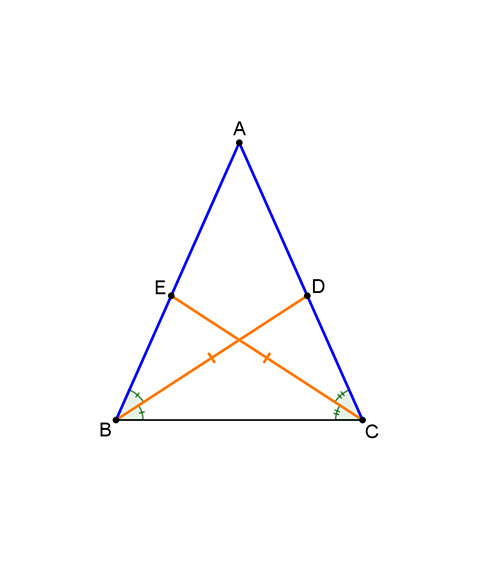# Just for the isosceles?

Geometry Level 2It is easy to see that if two sides of a triangle are equal (i.e. if the triangle is isosceles), then the corresponding angle bisectors are equal as well.

In a given triangle $ABC$ , $BD$ and $CE$ are the angle bisectors of $\angle B$ and $\angle C$ respectively.

If $\left| {\overline {BD} } \right| = \left| {\overline {CE} } \right|$, is it necessarily true that $\left| {\overline {AB} } \right| = \left| {\overline {AC} } \right|$?

Hint: Angle bisector theorem might be useful.

×